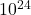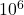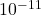A satellite that is in a circular orbit 230 km above the surface of the planet Zeeman-474 has an orbital period of 89 min. The radius of Zee

Question

A satellite that is in a circular orbit 230 km above the surface of the planet Zeeman-474 has an orbital period of 89 min. The radius of Zeeman-474 is 6.38 × 10 6 m. What is the mass of this planet?

in progress 0
5 months 2021-08-26T01:05:52+00:00 1 Answers 41 views 0

Mass of the planet = 6.0 ×Explanation:

Time period = 2π (R + h) / v

Orbital speed (v) = √GM / (R + h)

T² = 4π² (R + h)² / (GM/ (R + h))

= 4π² (R + h)³ / GM

making m the subject of the formula

m = 4π² (R + h)³ / GT²

= 4π² ( 6.38 ×+ 230 × 10³ )³ / ( 6.67 ×) × (89 × 60)²

= 4π² ( 6610000)³ / ( 6.67 ×) × (89 × 60)²

= 5.99 ×= 6.0 ×